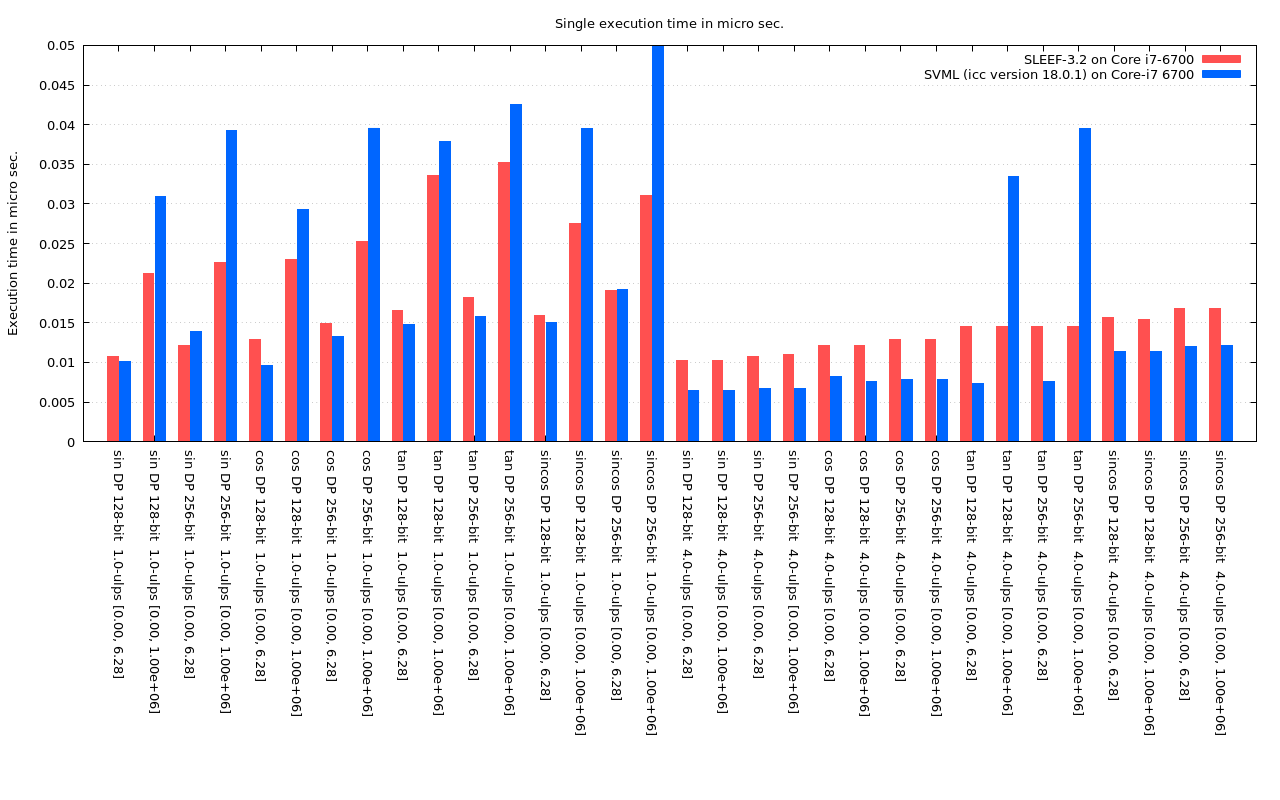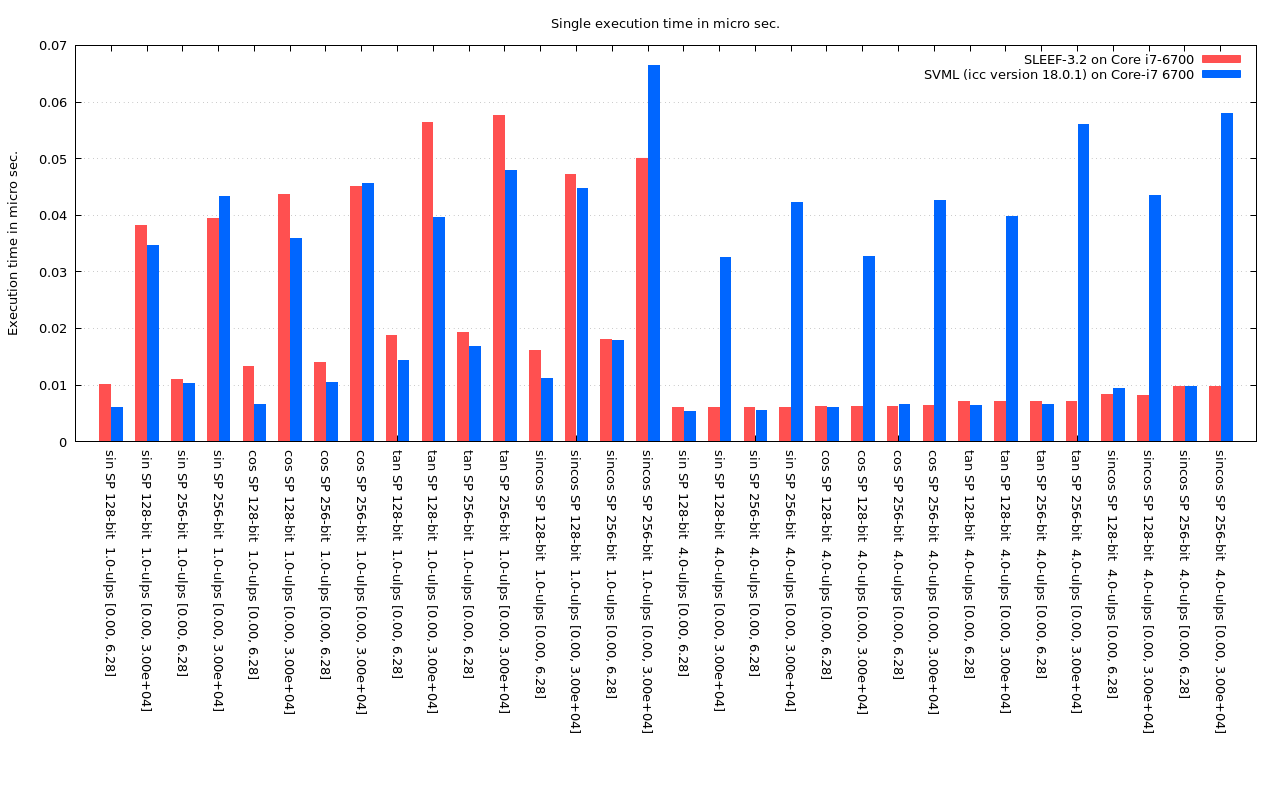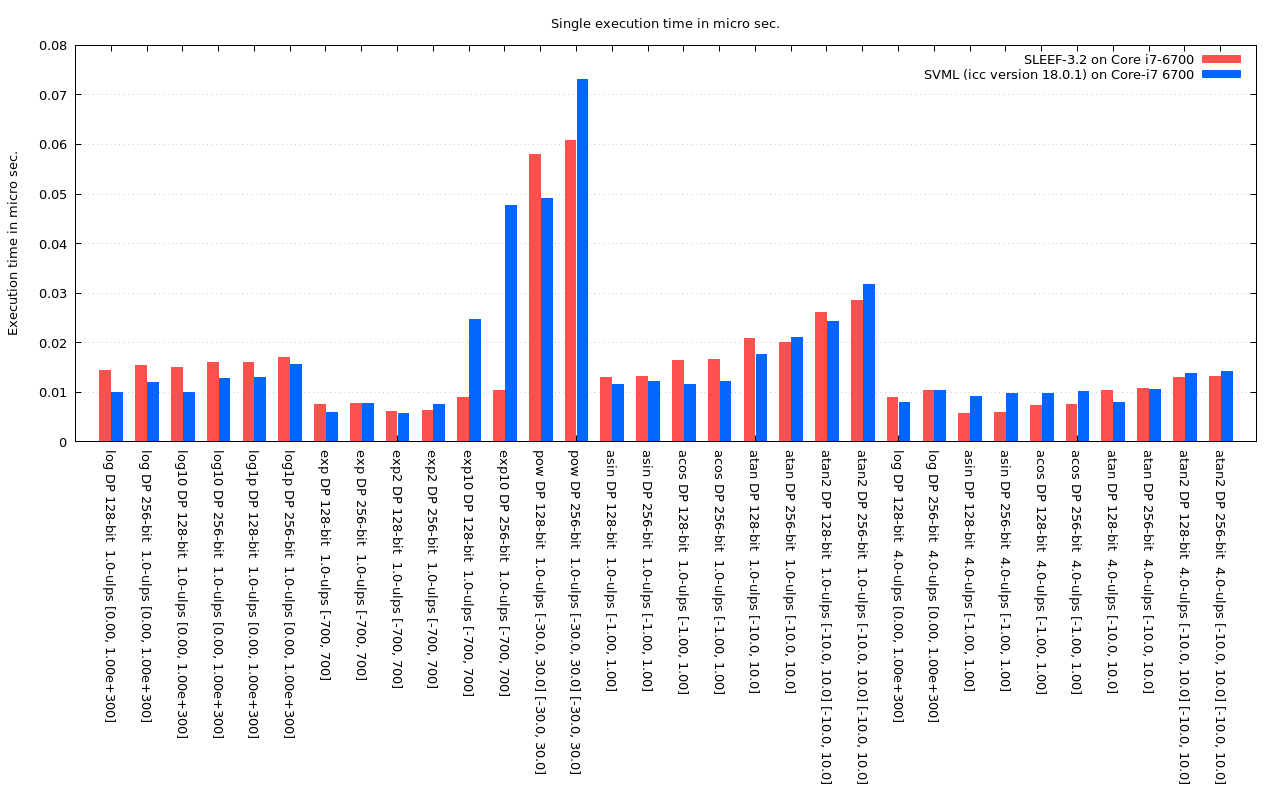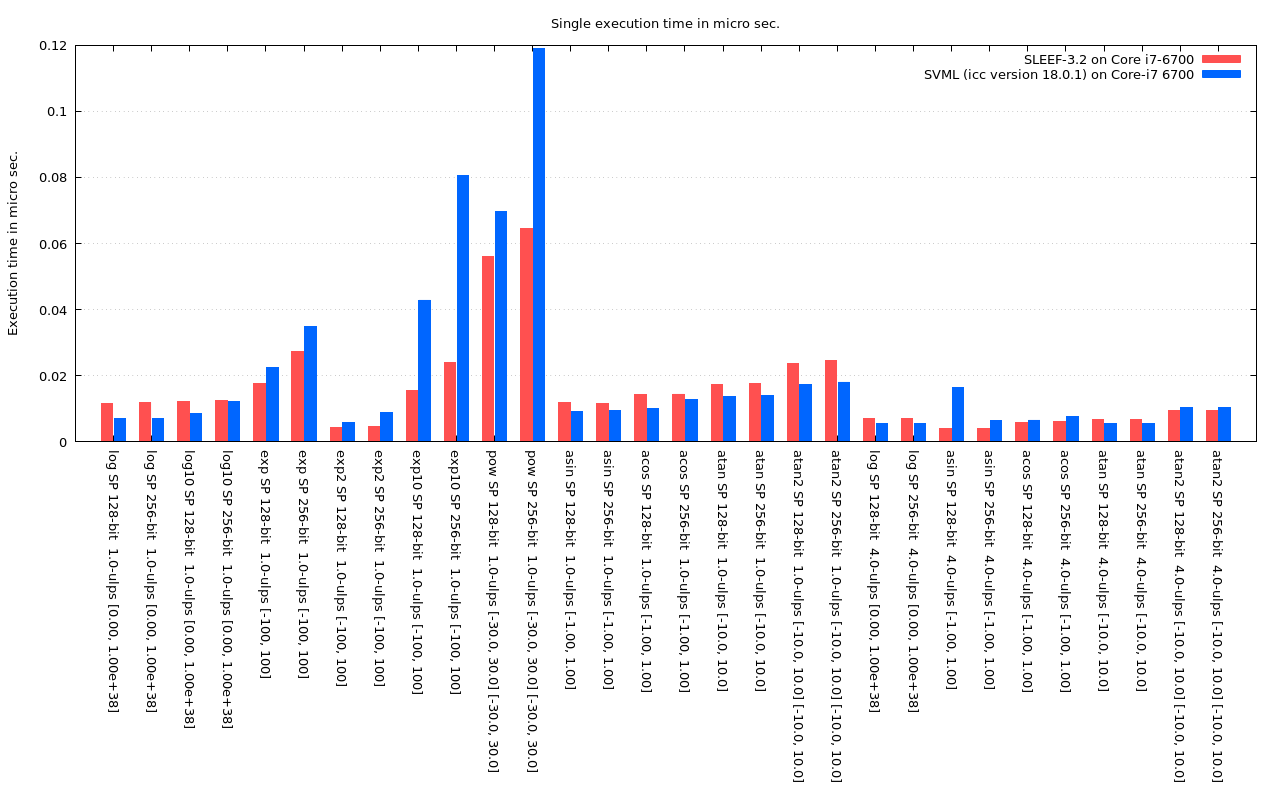# SLEEF - Benchmark Results

## Benchmark results

These graphs show comparison of the execution time between SLEEF-3.2 compiled with GCC-7.2 and Intel SVML included in Intel C Compiler 18.0.1.

The execution time of each function is measured by executing each function 10^8 times and taking the average time. Each time a function is executed, a uniformly distributed random number is set to each element of the argument vector(each element is set a different value.) The ranges of the random number for each function are shown below. Argument vectors are generated before the measurement, and the time to generate random argument vectors is not included in the execution time.

• Trigonometric functions : [0, 6.28] and [0, 10^6] for double-precision functions. [0, 6.28] and [0, 30000] for single-precision functions.
• Log : [0, 10^300] and [0, 10^38] for double-precision functions and single-precision functions, respectively.
• Exp : [-700, 700] and [-100, 100] for double-precision functions and single-precision functions, respectively.
• Pow : [-30, 30] for both the first and the second arguments.
• Asin : [-1, 1]
• Atan : [-10, 10]
• Atan2 : [-10, 10] for both the first and the second arguments.

The accuracy of SVML functions can be chosen by compiler options, not the function names. "-fimf-max-error=1.0" option is specified to icc to obtain the 1-ulp-accuracy results, and "-fimf-max-error=5.0" option is used for the 5-ulp-accuracy results.

Those results are measured on a PC with Intel Core i7-6700 CPU @ 3.40GHz with Turbo Boost turned off. The CPU should be always running at 3.4GHz during the measurement.

Click graphs to magnify.Fig. 6.1: Execution time of double precision trigonometric functionsFig. 6.2: Execution time of single precision trigonometric functionsFig. 6.3: Execution time of double precision log, exp, pow and inverse trigonometric functionsFig. 6.4: Execution time of single precision log, exp, pow and inverse trigonometric functions